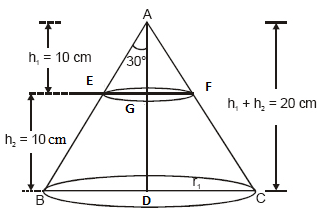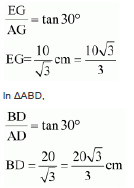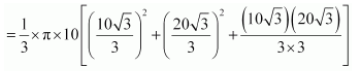Newbie

# A metallic right circular cone 20 cm high and whose vertical angle is 60° is cut into two parts at the middle of its height by a plane parallel to its base. If the frustum so obtained is drawn into a wire of diameter 1/16 cm, find the length of the wire. Q.5

• 0

Find the best solution of ncert class 10 chapter surface areas and volumes . Give me the best solution of the exercise 13.4 question no. 5 . Sir please give me the easiest and simplest  solution of this question. A metallic right circular cone 20 cm high and whose vertical angle is 60° is cut into two parts at the middle of its height by a plane parallel to its base. If the frustum so obtained is drawn into a wire of diameter 1/16 cm, find the length of the wire.

Share

1. The diagram will be as followsConsider AEGRadius (r1) of upper end of frustum = (10√3)/3 cm

Radius (r2) of lower end of container = (20√3)/3 cm

Height (r3) of container = 10 cm

Now,

Volume of the frustum = (⅓)×π×h(r12+r22+r1r2)Solving this we get,

Volume of the frustum = 22000/9 cm3

The radius (r) of wire = (1/16)×(½) = 1/32 cm

Now,

Let the length of wire be “l”.

Volume of wire = Area of cross-section x Length

= (πr2)xl

= π(1/32)2x l

Now, Volume of frustum = Volume of wire

22000/9 = (22/7)x(1/32)2x l

Solving this we get,

l = 7964.44 m

• 0